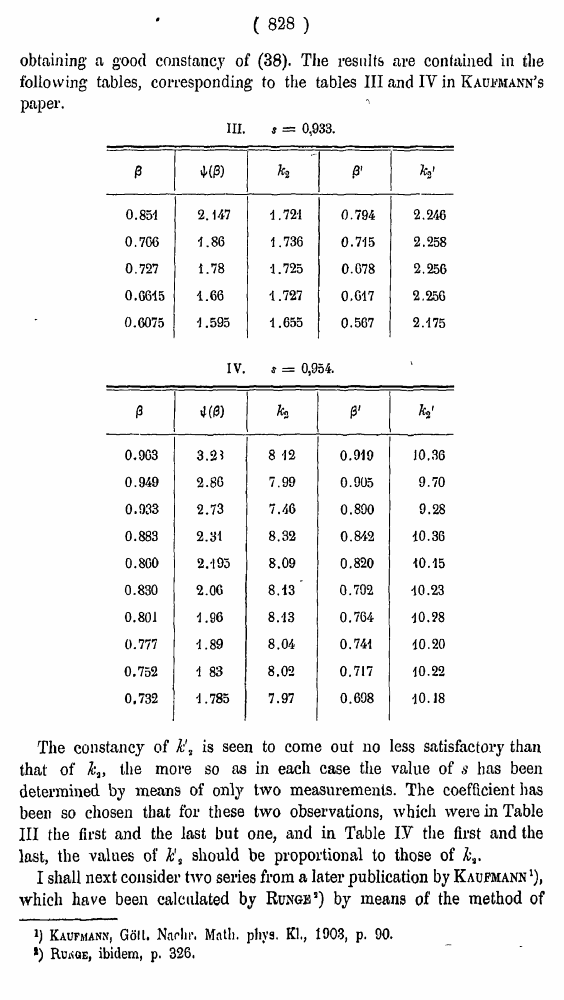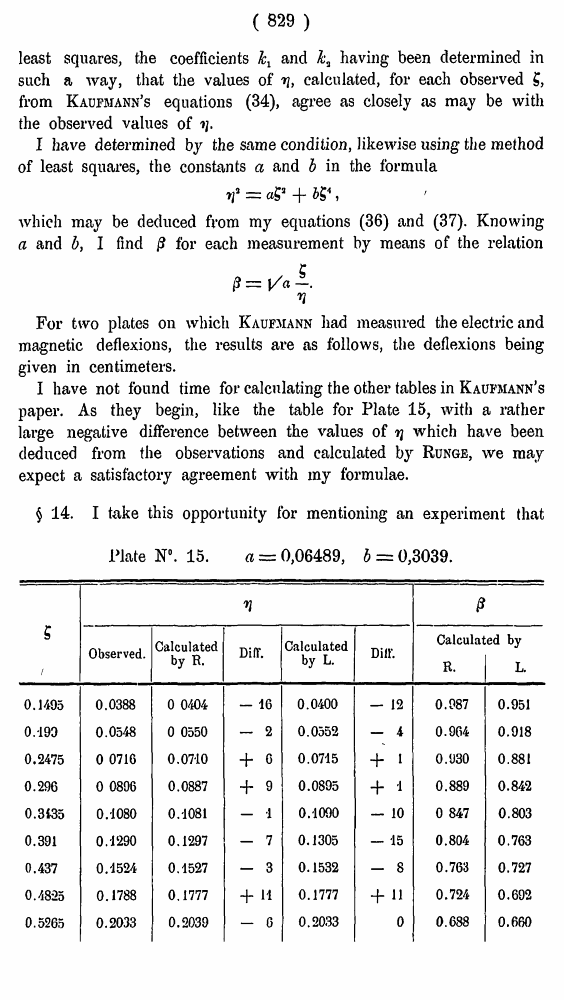# H. A. Lorentz. Electromagnetic phenomena in a system moving with any velocity smaller than that of light. // Proceedings Royal Acad., Amsterdam. Vol. VI., 1904

В начало   Другие форматы   <<<     Страница 828   >>>809  810  811  812  813  814  815  816  817  818  819  820  821  822  823  824  825  826  827  828 829  830  831

( 828 )

obtaining a good constancy of (38). The results are contained in the following tables, corresponding to the tables III and IV in Kaufmann's paper.

III. s = 0,933.

 β ψ(β) k2 β' k'2 0.851 2.147 1.721 0.794 2.246 0.766 1.86 1.736 0.715 2.258 0.727 1.78 1.725 0.078 2.256 0.6615 1.66 1.727 0.617 2.256 0.6075 1.595 1.655 0.567 2.175

The constancy of is seen to come out no less satisfactory than that of k3, the more so as in each case the value of s has been determined by means of only two measurements. The coefficient has been so chosen that for these two observations, which were in Table III the first and the last but one, and in Table IY the first and the last, the values of h's should be proportional to those of I shall next consider two series from a later publication by Kaufmann1), which have been calculated by Runge5) by means of the method of

Kaufmann, Goll. Nadir. Matli. phys. Kl., 1903, p. 90.

*) Runge, ibidem, p. 326.

IV. * = 0,954.

 β ψ(β) k2 β' k'2 0.903 3.2? 8 -12 0.919 10.36 0.949 2.80 7.99 0.905 9.70 0.933 2.73 7.46 0.890 9.28 0.883 2.31 8.32 0.842 10.36 0.800 2.-193 8.09 0.820 10.15 0.830 2.06 8.13' 0.702 10.23 0.801 1.96 8.13 0.764 10.98 0.777 1.89 8.04 0.741 10.20 0.752 1 83 8.02 0.717 10.22 0,732 -1.785 7.97 0.698 10.18

( 829 )

least squares, the coefficients and k3 having been determined in such a way, that the values of v, calculated, for each observed £, from Kaufmann’s equations (34), agree as closely as may be with the observed values of •»;.

I have determined by the same condition, likewise using the method of least squares, the constants a and b in the formula

r

which may be deduced from my equations (36) and (37). Knowing a and b, I find /? for each measurement by means of the relation

For two plates on which Kaufmann had measured the electric and magnetic deflexions, the results are as follows, the deflexions being given in centimeters.

I have not found time for calculating the other tables in Kaufmann’s paper. As they begin, like the table for Plate 15, with a rather large negative difference between the values of tj which have been deduced from the observations and calculated by Runge, we may expect a satisfactory agreement with my formulae.

§ 14. I take this opportunity for mentioning an experiment that

Plate N°. 15. a — 0,06489, b = 0,3039.

 V 0 s 1 Observed. Calculated by R. Diff. Calculated by L. Dill’. Calculat R. ,ed by L 0.1495 0.0388 0 0404 — 16 0.0400 — 12 0.987 0.951 0.190 0.0548 0 0550 — 2 0.0552 — 4 0.964 0.918 0.2475 0 0716 0.0710 + 6 0.0715 + 1 0.930 0.881 0.296 0 0896 0.0887 + 9 0.0895 + 1 0.889 0.842 0.3135 0.1080 0.-1081 — •1 0.1090 — 10 0 847 0.803 0.391 0.1290 0.1297 — 7 0.1305 — 15 0.804 0.763 0.437 0.4524 0.1527 — 3 0.1532 - 8 0.763 0.727 0.48-25 0.1788 0.1777 + 11 0.1777 + H 0.724 0.692 0.5265 0.2033 0.2039 — 6 0.2033 0 0.688 0.660